# Digging for topological property in disordered alloys: the emergence of Weyl semimetal phase and sequential band inversions in PbSe–SnSe alloys

Zhi Wanga, Qihang Liub, Jun-Wei Luoc and Alex Zunger*a
aRenewable and Sustainable Energy Institute, University of Colorado, Boulder, Colorado 80309, USA. E-mail: Alex.Zunger@colorado.edu
bShenzhen Institute for Quantum Science and Technology and Department of Physics, Southern University of Science and Technology, Shenzhen 518055, China
cState Key Laboratory for Superlattices and Microstructures, Institute of Semiconductors, Chinese Academy of Sciences, Beijing 100083, China

Received 16th April 2019 , Accepted 16th July 2019

First published on 16th July 2019

The search for topological systems has recently broadened to include random substitutional alloys, which lack the specific crystalline symmetries that protect topological phases, raising the question of whether topological properties can be preserved, or are modified by disorder. To address this question, we avoid methods that assumed at the outset high (averaged) symmetry, using instead a fully-atomistic, topological description of an alloy. Application to the (PbSe)1−x(SnSe)x alloy reveals that topology survives in an interesting fashion: (a) spatial randomness removes the valley degeneracy (splitting ≥150 meV), leading to a sequential inversion of the split valley components over a range of compositions; (b) the absence of inversion lifts spin degenerates, leading to a Weyl semimetal phase without the need of an external magnetic field, an unexpected result, given that the alloy constituent compounds are inversion-symmetric. (a) and (b) underpin the topological physics at low symmetry and complete the missing understanding of possible topological phases within the normal-topological insulator transition.

## New concepts

Topological properties are enabled and protected by symmetry. Yet, to realize these properties in real materials researchers have broadened databases to include alloys. Do topological properties survive if alloy disorder comes into play? In this work, we have demonstrated for substitutionally random alloys a new concept of a fully-atomistic, topological description, which can restore all local symmetry breaking effects due to alloy disorders, without assuming in advance an averaged or artificially high-symmetry structure. By using a combined strategy of a specially-designed supercell model, band unfolding mechanism and topological invariant calculation, we show the topological phase transition under alloy disorder at an atomic resolution, with all E vs. k band dispersion restored. Our concept goes beyond the ‘monomorphous’ alloy theory, which assumes a single local environment for all alloy atoms. For (PbSe)1−x(SnSe)x alloy, we find a Weyl semimetallic, sequential band inversion regime between normal insulator and topological crystalline insulator phases, even at zero external magnetic field, which is unexpected and can only be explained by the atomistic alloy disorder effect. Our findings expand the horizons for designing and predicting new physics, which originates not from pre-existed symmetries in building blocks but from the symmetry lowering by applying as a disordered alloy.

## Introduction

Topological physics offers the intriguing idea that certain properties at lower dimensions – such as 2D surface or 1D edge states of a 3D bulk crystal sample – can be gleaned from the understanding of certain bulk symmetries, rather than from the understanding of broken bonds at lower dimensions. Indeed, topological insulators (TI), topological crystalline insulators (TCI), topological Dirac semimetals (TDSM), and Weyl semimetals (WSM) are enabled by specific bulk band structure symmetries, e.g., time-reversal symmetry1,2 (for TI), mirror3 or nonsymmorphic4,5 symmetry (for TCI), and rotational symmetry6 (for TDSM), whereas for WSM it requires crossings with linear dispersion between two nondegenerate bands (Weyl points), and hence requires breaking of time reversal or inversion symmetry.

Experimental explorations of the properties of such topological phases, including chemical trends among different compounds, naturally require stable, synthesizable samples. Unfortunately, the number of such experimentally proven pure (non-alloyed) compounds with extraordinary topological properties appears at this time to be rather small. To raise the number of such possible compounds, density functional band structure theory7–9 has recently predicted additional compounds. For example,8 considering compounds that are TI with finite band gap ≥1 meV between the highest occupied and lowest unoccupied bands, revealed just 181 predictions from a starting database of 184270 materials in ICSD (89240 that have very accurate measured atomic structures were then picked up, from which 26938 ‘stoichiometric compounds’8 were finally inspected to find TI candidates). Given that such tiny-gap compounds are hardly insulators of experimental interest, the number of forecasted TI's with above thermal energy gaps is surely considerably smaller. Another frontier of TI materials is to find topological states in antiferromagnets, where both time reversal and primitive-lattice translational symmetries are broken, but the combination of the two is preserved.10 This antiferromagnetic TI was not found until recently, as Otrokov et al.11 reported that MnBi2Te4 film could be the first example. Moreover, the recent discovery that many of the predicted TI's are false positive,12 further suggests that one can expect indeed only a small number of stable, synthesizable, non-alloyed compounds that are good TI candidates.

The disappointingly small projected number of gapped TI's illustrates the reason behind numerous recent attempts, to broaden the material database of various topoloid systems by mixing pure compounds, making substitutional disordered alloys. Examples include BixSb1−x13–15 (a TI), or (PbSe)1−x(SnSe)x16,17 (a TCI), or (Na3Bi)x(Na3Sb)1−x18 (a TDSM) or MoxW1−xTe219 (a WSM). An alloy provides an external “knob” to manipulate the topological phase transition by an order parameter, and hence provides insights about the emergent physical phenomena. However, given that the topological designation is intimately dependent on the crystalline bulk band structure symmetry, there are central questions that remain unsolved: how does alloy disorder affect bulk symmetries, and how will the ensuing symmetry changes in the alloy affect its topological properties and the classes of topology?

These questions are often overlooked in the theoretical literature, because it is sweepingly accepted in standard models of disorder that the observable property can be calculated as the property P(〈S〉) of the alloy-averaged structure 〈S〉, rather than the averaged property 〈P(Si)〉 of many individually local-symmetry-breaking (low-symmetric) alloy configurations {Si}. For instance, in the virtual crystal approximation20 (VCA), it is envisioned that a substitutional, perfectly random alloy should maintain the high symmetries as in their underlying, non-alloyed constituent components;16,21 the single-site coherent potential approximation18,22,23 (S-CPA) makes a better approach, distinguishing the two alloyed sublattices A and B, but assuming that all A sites (and separately all B sites) experience a single effective potential, irrespective of the nature of its coordination by different combinations of A and B sites. In such monomorphous approaches, it is assumed that a single structural motif tiles the entire alloy, so the alloy symmetry in perfectly random, large samples should equal that of the constituent, non-alloyed compounds. While this may be true for the macroscopically averaged alloy configuration S0 = 〈Si〉, it need not be true for any particular realizations of randomly occupying the N lattice sites by A and B atoms. Such realizations create a polymorphous network, consisting of individual configurations {Si}, where any of the atomic sites can be surrounded locally by a different number and orientations of A–A, A–B, and B–B bonds, and such local configurations can have not only (i) different symmetries, but also manifest (ii) different degrees of charge transfer with respect to the neighbors, as well as (iii) different A–A, B–B, and A–B bond lengths, all of which constitute symmetry lowering. As a result, the physical properties P(Si) of such individual, low symmetry random realizations, can be very different (e.g., have splitting of degenerate levels) from the property of the fictitious, high symmetry macroscopically averaged configuration P(S0), and thus the observed physical property 〈P〉 will then be the average of the properties 〈P(Si)〉, but not P(S0).

A natural approach that captures such polymorphous aspect of random alloys is the supercell approach, where lattice sites are occupied by the alloyed elements with a particular form of disorder and the system is solved via periodic electronic structure methods. However, such approach tends to produce complex E versus k dispersion relations, making the analysis of topological properties that closely depend on the wave vector concept cumbersome. A solution that retains the polymorphous nature of the random alloy but reinstates the E versus k relation in the base Brillouin zone is to unfold the supercell bands.24–26 This yields the alloy “effective band structure” (EBS), providing a three-dimensional picture of the distribution of spectral density in the whole Brillouin zone. In a recent work27 we have applied such EBS method on the supercell approach, and examined the trends of various strengths of disorder in a few cases, including CdTe–HgTe, PbSe–SnSe, and PbS–PbTe alloys. In CdTe–HgTe, the disorder effects were so weak that the monomorphous approaches were still feasible. In PbSe–SnSe, the stronger disorder effect introduces significant (∼150 meV) band splitting of the topological band inversion. In PbS–PbTe, there is a strong disorder effect, revealing the emergence of ferroelectricity from the polymorphous network in this alloy.

In the present paper we address the question of the consequences of alloy disorder on the problem of WSM topology. WSM phase has recently attracted significant attention19,28–31 because exotic physics has emerged from its surface electronic structures (e.g., Fermi arcs) and responses to external magnetic fields (e.g., chiral anomaly). A WSM has band crossings at Weyl points with linear dispersion between two non-degenerate bands (see Fig. 1d), and thus requires breaking of time reversal or inversion symmetry. Recently,14,32 a WSM phase has been predicted to exist by tuning an order parameter (such as strain) between noncentrosymmetric NI and TI phases. However, such previous predictions suggest that the origin of the Weyl phase is the broken inversion symmetry that exists already in the alloy constituents, such as MoTe2 and WTe2 in the (MoTe2)x(WTe2)1−x.19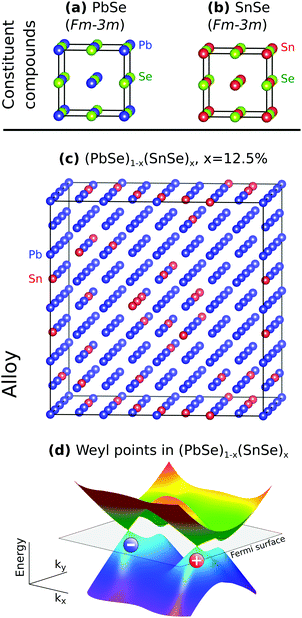Fig. 1 Atomic structures of (a) pure PbSe, (b) pure SnSe, (c) alloy (PbSe)1−x(SnSe)x, all in cubic phase, and (d) a schematic plot for Weyl points in such alloy. (c) is one supercell realization from the many special quasirandom structure (SQS) realizations. Se atoms are not shown in (c). The red and blue balls in (d) show the chirality of the two Weyl points.

We consider the topological phases of the cubic-phase (PbSe)1−x(SnSe)x alloys, characterized by the 8-fold band edge states of the constituents. Using this alloy as an example, we show how our method gives a picture of topological phase transition under alloy disorder in an atomic resolution, and how it answers the question of disorder effects on topological properties and the classes of topology in an alloy system. Our method reveals that:

(a) Instead of finding a concurrent transition between normal insulator and TCI phases, as predicted using standard alloy models16,18,21,23 that artificially retain the high symmetry of components, we find a new, sequential, one-by-one inversion of the split L-valley components (by as much as 150 meV) over a range of alloy compositions. A sequential transition occurs between the insulating alloy and a metallic phase in (PbSe)1−x(SnSe)x over a finite range of compositions (here, 12% < x < 30%). This is consistent with an optical experiment33 that observes a composition range (13% < x < 24%) for the transition (however, a gap smaller than 50 meV could not be detected). Perhaps in future, high-resolution experiments e.g. low-temperature THz range optics and Angle-Resolved Photoemission Spectroscopy (ARPES) could narrow down the range.

(b) The removal of spin degeneracy by lifting inversion symmetry in the alloy leads to a WSM phase (the separation of Weyl points in momentum space can be larger than 0.05 Å−1) in the sequential band inversion regime, even without the need of an external magnetic field (Fig. 1d).

Both realizations (a) and (b) originate from the local symmetry breaking. The fact that both PbSe and SnSe in the cubic phase have inversion-symmetry, yet a Weyl phase is predicted in random alloy (PbSe)1−x(SnSe)x, is unprecedented, and implies that the effect owes its existence to inversion symmetry breaking induced by alloy disorder, not pre-existing in the constituent compounds. A recent observation34 of linear magnetoresistance in (PbSe)1−x(SnSe)x alloy in very small magnetic field reveals the symmetry breaking in charge density, which agrees with the above conclusion. High-resolution experimental probing of our predictions for sequential band inversion and Weyl points is required. While it has been customary to identify WSM in structural types with broken time-reversal symmetry or broken inversion symmetry,35 the realization of Weyl phase from non-magnetic, inversion-symmetric building blocks may clarify an important but missing piece in the puzzle of new topological materials.

## Results

### Symmetry and topology in substitutional random alloys

Substitutional (PbSe)1−x(SnSe)x alloys have been found long ago to show band inversion.33 The alloy is cubic at low Sn composition, but then has a cubic–orthorhombic transition at Sn > 45%; the topological transition, whereby the cation-like conduction band minimum (CBM) and the anion-like valence band maximum (VBM) swap their order is observed around Sn = 20% i.e. before the cubic-orthorhombic transition.36 In Fig. 1 we show the cubic phase atomic structures for this alloy, together with its two constituent compounds PbSe and SnSe.

What makes this system particularly interesting in the current context is the band edges (CBM and VBM) involved in band-inversion (four L points) and a twofold Kramers-degeneracy (at each L). Fig. 2 illustrates different views on the topological transition in this alloy system. Here the cation-like, L6 symmetric CBM (labeled C1–C4) is shown as blue and the anion-like, L+6 symmetric VBM (A1–A4) as red. The system is a normal insulator (NI) when the cation-like states are above the anion-like states (blue-above-red); otherwise, the system is a band-inverted TCI (red-above-blue). There are two possible scenarios for the topological transition: If the valley degeneracy of the L point is preserved in the alloy (Fig. 2a and b), as assumed in e.g. VCA and S-CPA, the NI–TCI transition will involve all degenerate members becoming band inverted in tandem, as a concurrent transition, and the system becomes a zero gap metal at just a single composition (Fig. 2b).16,18,21,23 Fig. 2e and f illustrate, respectively, the VCA and S-CPA concurrent band inversions. If, on the other hand, alloy randomness splits the degenerate states, the transition occurs sequentially as shown in Fig. 2c and d and extends over a range of compositions (Fig. 2d). Fig. 2g and h illustrate the sequential transition as found in the atomistic supercell description. Obviously, theoretical alloy methods assuming that the configurationally averaged symmetry is S0 = 〈Si〉 predict a sharp transition between NI and TCI as illustrated in Fig. 2a and b for the (PbSe)1−x(SnSe)x and (PbTe)1−x(SnTe)x family.16,18,21,23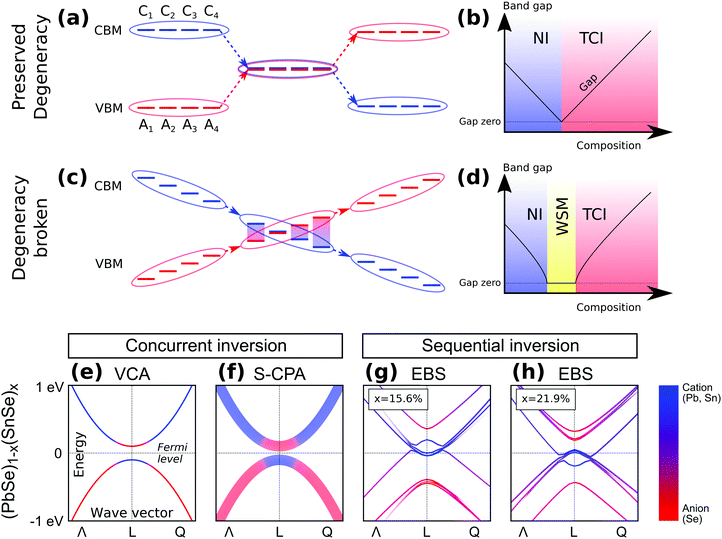Fig. 2 Two topological transition diagrams for (PbSe)1−x(SnSe)x alloy. (a) and (b): (a) the ‘concurrent scenario’ where the L valleys stay degenerate and the transition is concurrent; (b) the topological phase evolution with composition for the concurrent scenario. (c) and (d): (c) the ‘sequential scenario’ where randomness splits the valley degeneracy leading to sequential band inversion at L point; (d) the topological phase evolution corresponding to sequential scenario. The bottom row shows the alloy band structures from (e) VCA (schematic), (f) S-CPA (schematic), and (g) and (h) effective band structures (EBS) from two 256-atom SQS supercells (Sn = 15.6% and Sn = 21.9%).

One expects that in general a random substitutional alloy will be inherently polymorphous, i.e., each of the A sites ‘see’ a different potential (and so do each of the B sites). Whether this causes a measurable breaking of valley degeneracy depends on the delocalization vs. localization of the pertinent wavefunction, which in turn reflects the physical disparity (size; electronic properties) between the two alloyed elements A and B. We find in (PbSe)1−x(SnSe)x a significant (≥150 meV) breaking of degeneracy, leading to two consequences. (a) Band inversion can happen sequentially (one-by-one), creating a metallic phase between the NI and TCI phases over a range of alloy compositions (Fig. 2d). (b) We identify a WSM phase in the metallic regimes, which originates from the disorder-induced inversion symmetry breaking in the polymorphous network.

### Atomistic alloy theory with different scales of disorder

That the macroscopically observed property 〈P〉 is the average of the properties {P(Si)}, not the property of the average configuration P(S0) is evident, among others, from the fact that local probes generally observe symmetry broken structures. Examples include the extended X-ray absorption fine structure (EXAFS) measurements of A–X and B–X bond lengths in numerous substitutional alloys such as GaAs–InAs, PbTe–GeTe, ZnTe–CdTe and PbS–PbTe,37–41 and the successful simulation of such observations on the basis of strain minimization.42 Similarly, whereas measurements that have an intrinsically large coherence length such as X-ray diffraction tend to produce high symmetry structures when the data is fitted to small unit cell structures, more discriminating probes such as the atomic pair distribution function (PDF) show alloy bond alternation and length distributions,43,44 i.e., R(A–X) ≠ R(B–X).

To analyze the distinct physical contributions to alloy formation we consider three conceptual steps. First, compress the component having a larger volume and expand the one with the smaller volume, so that both can fit into the coherent alloy structure cell (here, Vegard's volume V(x) for (PbSe)1−x(SnSe)x). Fig. 3a shows that this “volume deformation” step changes linearly the gap of one component (here, SnSe) while creating a V-shape gap in another component (here, PbSe). Second, mix the two prepared components onto the alloy lattice at fixed volume V(x), allowing charge rearrangement between the alloyed components, reflecting their possibly different Fermi levels. This is shown in Fig. 3b by the self-consistent density functional theory (DFT) charge density plot in a randomly created alloy supercell. One notices a non-negligible difference in the density along the Pb–Se bond relative to the Sn–Se bond. Finally, given that the alloy manifests a range of different local environments (e.g., the common atom Se can be locally coordinated by different metal atoms PbNSn6−N with 0 ≤ N ≤ 6), this can cause atomic displacements, illustrated here by the DFT calculated different Sn–Se and Pb–Se bond lengths at each alloy lattice constant (even if the latter follows Vegard's rule). This is illustrated in Fig. 3c indicating that in the alloy environment the Pb–Se bond is distinct from the Sn–Se bond and both are different from Vegard's average. We expect that the scale of disorder in this alloy is at an intermediate level (PbSe–SnSe mismatch of 3.5%), if compared to (CdTe)x(HgTe)1−x (weakly disordered; size mismatch of CdTe–HgTe is 0.3%) and (PbS)x(PbTe)1−x (strongly disordered; size mismatch of PbS–PbTe is 7.9%). The respective electronegativity difference between Pb and Sn is 0.09 (0.31 for Hg–Cd and 0.48 for S–Te).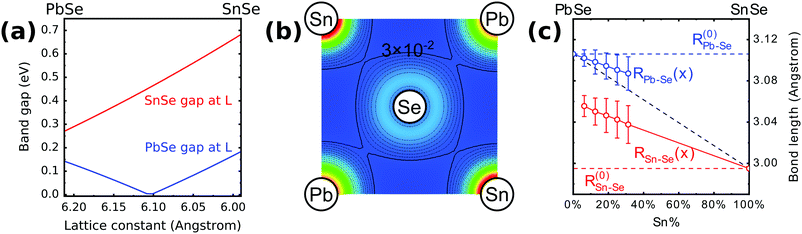Fig. 3 DFT results for the three leading alloy effects illustrated for (PbSe)1−x(SnSe)x. (a) Band gaps at the L point of PbSe (blue) and SnSe (red) under different lattice constants, (b) total charge density in (PbSe)1−x(SnSe)x alloy created from equal volume components, and (c) bond length distribution of Pb–Se and Sn–Se (blue and red solid line with bars as the standard deviations) compared with their values in pure compounds (blue and red dash lines) and the Vegard's rule (black dash line), under different Sn compositions.

Monomorphous models such as VCA and S-CPA account for volume deformation but rely on the assumption that alloy charge exchange disorder or atomic displacement disorder has negligible effects (S-CPA considers approximately the former but neglects the later, while VCA neglects both). Other widely used single-site disorder methods such as the tight-binding effective Hamiltonian add a random on-site potential εi ∈ [−W,W] to the diagonal energy in the Hamiltonian,45 predicting topological Anderson insulator (TAI),45,46 while neglecting off-site disorder (such as charge transfer and different bond lengths as shown in Fig. 3b and c) and the existence of a distribution of local symmetries.

### Achieving atomic resolution for alloys in supercells

To achieve atomic resolution of disorder one needs theory that recognizes local symmetry yet informs about the extent to which the long-range translational symmetry is retained. Such atomistic theory of alloys has been previously achieved via supercells where each Ai site (i = 1…N) has in principle a different local environment and the same for each Bj site, forming a polymorphous network of many atomic local environments. A specially constructed Special Quasirandom Structure (SQS)47,48 provides the best choice in guaranteeing the best match of correlation functions of the infinite alloy possible for the N atom supercell, where N is increased until the required properties converge. A brief introduction of SQS has been given in the Methods.

However, while supercells can directly include the atomistic effects discussed above, it folds all bands and thus results in an ensuing complex ‘band structure’ which is difficult to interpret as it lacks a wavevector representation (Fig. 4a). Not surprisingly, the results of supercell calculations were most often presented as DOS, thus giving up the ability to recognize topological characteristics that are wavevector dependent. This absence of a relevant E vs. k dispersion relation can be solved by unfolding the bands into the primitive Brillouin zone (BZ). This results in an alloy Effective Band Structure (EBS)24–26 with a 3-dimensional spectral function distribution for each alloy band. Similar to angular resolved experimental spectroscopy, EBS consists of both coherent (dispersive term e.g. bands at L0 – one of the 4 L points in Fig. 4b and c) and incoherent (non-dispersive broadening e.g. VBM at W point in Fig. 4b) features, which emerges naturally from all disorder effects allowed in the supercell. With the E vs. k dispersion restored by EBS, it can be determined then if topological features are retained or destroyed. The basic concept of EBS has been given in the Methods.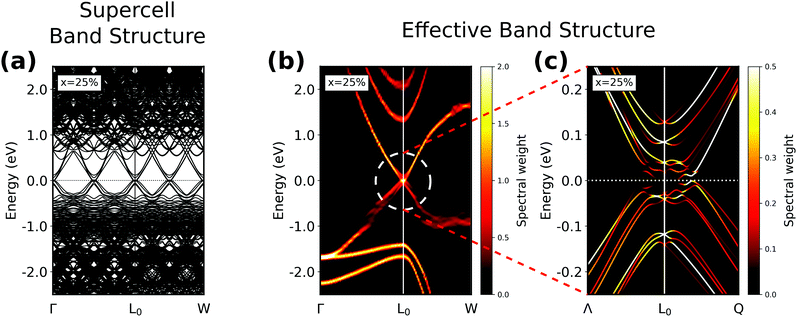Fig. 4 Comparison between supercell band structure and EBS in a (PbSe)1−x(SnSe)x SQS supercell. (a) Supercell band structure (256-atom supercell (PbSe)1−x(SnSe)x at x = 25%, plotted in the primitive BZ of Fm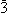m PbSe primitive cell). (b) EBS (unfolded from 256-atom supercell (PbSe)1−x(SnSe)x at x = 25% into the same primitive BZ as in (a)). (c) The same EBS as in (b) but zoomed-in around the L0 point (one of the 4 L points). (a–c) are all plotted along the same γ–(λ)–L0–(q)–W direction in the primitive BZ (λ = (0.875π/a, 0.875π/a, 0.875π/a), L0 = (π/a, π/a, π/a), q = (π/a, 1.0625π/a, 0.9375π/a) and W = (π/a, 1.5π/a, 0.5π/a)). As shown in (c), there are two types of splitting: valley degenerates’ splitting at the L0 point (at the vertical white solid line) which is about 150 meV, and the spin degenerates’ splitting (rashba-like) around L0 which is 10–30 meV.

### Breaking of valley degenerates causes a sequential, one-by-one inversion of the disorder-split bands

We generated multiple SQS supercells for Sn composition x in (PbSe)1−x(SnSe)x from 0% (pure PbSe) to 31.25% to cover the NI–TCI transition regime without cubic-orthorhombic transition.36 We found that the CBM and VBM at the L points are not 4-fold valley-degenerate as predicted by the monomorphous approaches, instead they split and form 8 states (C1–C4 and A1–A4 as marked in Fig. 2c) nearby the Fermi level at the L point with a splitting energy of ≥150 meV, which reveals a significant loss of degeneracy on high-symmetry k points (here, L points). It shows that in this alloy the wavefunction indeed feels the alloy disorders, and the single band picture from the monomorphous description is inadequate. The splitting of degenerates in (PbSe)1−x(SnSe)x has been shown in Fig. 4c.

We then made a statistical analysis among different SQS (grouped by the Sn composition) on the eigen energies of the 8 split bands at L, which is shown in Fig. 5. We found that the 8 bands have relatively stable valley splitting and small overlap, making the band inversion at the L point a sequential process, i.e., at low composition the band inversion firstly occurs between C4 and A1, then with composition increasing the other bands become inverted one-by-one. This sequential inversion regime emerges from the lifting of the valley degeneracy at L by the alloy disorder. Therefore, this regime is not observable in monomorphous approaches, where the NI–TCI transition is sharp and concurrent.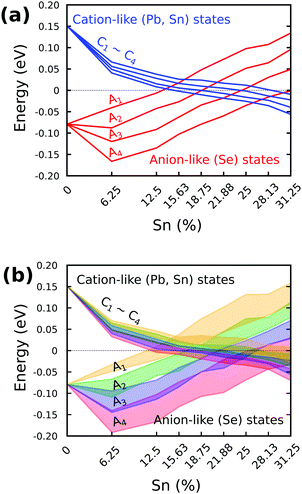Fig. 5 Sequential band inversion and band broadenings in (PbSe)1−x(SnSe)x supercells. (a) The averaged eigen values of the 4 cation-like (blue, C1–C4) and 4 anion-like (red, A1–A4) band branches from EBS at the L point in the primitive first BZ at different Sn compositions, calculated statistically from 160 SQS supercells (all are 256-atom supercells). (b) The standard deviations of the eigen values of C1–C4 and A1–A4, calculated statistically from 160 SQS supercells.

The band gap always locates between the 4th and 5th bands of the 8 split bands, however because of the one-by-one band inversion (e.g., at some composition the 4 unoccupied bands are C1, A1, C2 and A2, while the 4 occupied bands are C3, A3, C4 and A4), the band gap in this regime is very small and hence can be absent (beyond the measurable range of equipment)33 in measurement. In an optical experiment,33 the positive-gap (normal insulating) composition range was x < 10%, and the composition range where gap <50 meV (the detection limit) was 13% < x < 24%. Our results suggest a sequential band inversion in the composition range of 12% < x < 30%. Besides a possible DFT error, the comparison between our method and the experiment could also be affected by (1) the absence of band gap closing points in the experiment (the instrument could not measure the gap in the far-infrared region with IR detectors at the time of the experiment), and (2) non-randomness effect and high carrier concentration in experimental samples. Nevertheless, the existence of the composition range over which the transition occurs, and the stable splitting energy suggest that this sequential inversion regime might be observable in high-resolution experiments e.g. low-temperature THz range optics and Angle-Resolved Photoemission Spectroscopy (ARPES).

### Broken inversion symmetry due to alloy disorder leads to WSM phase in the sequential band inversion regime

The complex band crossing shown in Fig. 4 and 5 inspired us to study the topological property hidden inside the sequential band inversion regime. By calculating the band gap, we found that the polymorphous approach predicts metallic phase (bulk gap equals to zero) in the regime of the sequential band inversion process, where the four C1–C4 and the four A1–A4 have crosses among each other (12% < x < 30%). Within this metallic phase the mirror Chern number does not apply to characterize NI or TCI transition. We found that (PbSe)1−x(SnSe)x alloys have bulk Weyl points in this sequential band inversion regime, which drives the system to a WSM. The Weyl points originate directly from the breaking of local inversion symmetry, which is attributed to two reasons: (a) the atomic potentials are different between Pb and Sn, and (b) the atomic displacements are polymorphous and non-uniform. The intensity of inversion symmetry broken can be seen from the spin degenerates’ splitting (10–30 meV) around the L point, as shown in Fig. 4c. Note that previous works indicated that Weyl phases can appear between NI and TCI or TI phase,49,50 however they used either an external magnetic field or non-centrosymmetric compounds, thus the time reversal symmetry or inversion symmetry has been broken by an external knob or already in the selected building blocks. The conclusions in previous works are hence not applicable to our system, where the constituent compounds are both time-reversal and inversion symmetric.

By choosing different sizes and different space groups of the alloy supercell, we systematically look into the emergent phases in the band version regime. The Sn composition of each size of supercell has been fixed to 25%. We then use only uniform hydrostatic pressure, i.e., changing lattice constant continuously, as an order parameter to tune the band inversion and thus the NI–TCI topological transition in each supercell. Note that the motivation to change the lattice constant is to simulate the phase diagram with the concentration of Se. Łusakowski et al.51 predicted a metallic phase in (PbTe)1−x(SnTe)x alloy, also using uniform hydrostatic pressure as the order parameter, where they found many ‘jumps’ of the topological invariant as a function of order parameter in a 16-atom highly symmetric supercell. They then predicted that this metallic phase would also exist in larger 64- and 216-atom supercells (about 1000 configurations in total). Unfortunately, they did not examine if the metallic phase is topological, or symmetry- or size-related.

The flow chart for the recognition of topological properties in our systems has been given in the Method section (Fig. 8). The results have been listed in Table 1. We started from the highly symmetric, 8-atom supercells (space group Pmm), and removed symmetries from the supercell step by step, by enlarging the size and using atomic displacements (from Pmm to Amm2, to Pmm2, to PM, to P1). We found that (1) the metallic, sequential band inversion regime exists in every supercell we calculated, and (2) the zero bulk band gap always occurs on points or lines in momentum space. Furthermore, these gap-zero points or lines have symmetry-related properties: in the 8-atom supercells (Pmm), gap-zero points are Dirac points, protected by the inversion symmetry; removing symmetries step by step drives the gap-zero points from Dirac points to nodal-lines (Amm2), and finally to Weyl points (Pmm2, PM, and P1). Note that the P1 symmetry supercells are no longer TCI (due to the broken of mirror plane), but they still have the WSM phase in the sequential inversion regime.

Table 1 The summary of topological phase transition in different supercells of (PbSe)0.75(SnSe)0.25. We show in the first row the prediction from VCA and S-CPA as a comparison
Method Space group SG index No-inversion regime Sequential band inversion regime Full-inversion regime
VCA, S-CPA Fmm (2 atoms) 225 NI No such regime TCI

Supercells Pmm (8 atoms) 221 NI Dirac semimetal TCI
Amm2 (8 atoms) 38 NI Nodal-line semimetal TCI
Pmm2 (24 atoms) 25 NI WSM TCI
Pm (48 atoms) 6 NI WSM TCI
P1 (32 atoms) 1 NI WSM NI

We further showed the Weyl points in momentum space in Fig. 6: we chose the supercells having 24 atoms and space group Pm (lowest symmetry that can be TCI) at different lattice constants, then plotted the shortest distance of Weyl points that have opposite chirality (‘Weyl pair’), as well as the trajectories of Weyl points, as variables of lattice constant. We observed four Weyl points in this kind of supercell, forming two Weyl pairs. The separation between a Weyl pair can be larger than 0.05 Å−1, which is equal to the predicted value in (PbSe)1−x(SnSe)x alloy with external magnetic field.49 Moreover, the sequential band inversion regime (marked in yellow in Fig. 6a) always has non-zero separation between Weyl pairs. This illustrated that the WSM phase exists not accidently but in a wide range within the sequential band inversion range.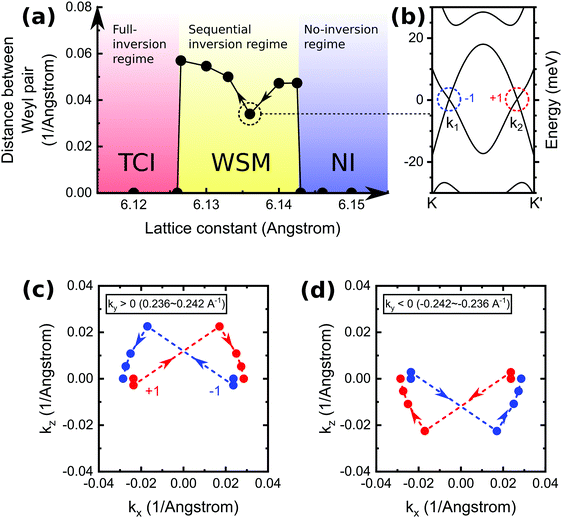Fig. 6 The separations and trajectories of Weyl points in k-space in (PbSe)0.75(SnSe)0.25 supercells. All supercells are 24-atom with space group Pm (mirror plane is (1−10)). (a) The shortest distance in k-space between Weyl points having opposite chirality, as a variable of lattice constant. (b) One of the two Weyl pairs along the k–k′ line (lattice constant = 6.136 Å), where k = (0.6614, 0.6975, 0.5345) and k′ = (0.6975, 0.6614, 0.5345) (cartesian coordinates along , , , unit in Å−1); the two Weyl points are at k1 = (0.6674, 0.6915, 0.5345) and k2 = (0.6915, 0.6674, 0.5345) (cartesian coordinates along , , , unit in Å−1) and marked by blue (chirality −1) and red (+1) circles. (c and d) The trajectories of the two Weyl pairs at different lattice constants; the directions of kx, ky and kz in (c) and (d) are [1−10],  and  directions, respectively; the origin points (0, 0, 0) Å−1 in (c) and (d) are L point; red and blue circles in (c) and (d) are Weyl points with chirality +1 and −1, respectively. The arrows in (c) and (d) are corresponding to the arrows in (a).

The appearance of Weyl phase in the sequential inversion regime explains the unusual oscillation in the spin Chern number noted by Łusakowski et al.51 Furthermore, a recent observation34 of linear magnetoresistance in (PbSe)1−x(SnSe)x alloy in very small magnetic field also reveals the symmetry breaking in charge density. We show that Weyl phase can appear in alloys even with its components having both time-reversal and inversion symmetries, which cannot be predicted if one assumes that the random alloy always restores the symmetry of constituent compounds. Such Weyl phase originates from the local symmetry breaking induced by disorder, which agrees with the magnetoresistance experiment and reveals the important role of the polymorphous network in the topological transition. For a random alloy, it is expected that different local configurations with disorder-induced symmetry breaking manifest Weyl points forming a spot rather than a point in the momentum space. We also expect that other alloy systems, e.g., halide perovskites ABX3, with bigger size mismatch between atoms will have larger atomic displacements, cause larger removal of inversion symmetry, and hence have Weyl points easier to be measured from experiments.

## Conclusions

Using a polymorphous network via a supercell representation that affords different local environments, followed by band unfolding and calculation of topological invariants, we accomplish a fully-atomistic picture of how topology survives alloy disorder. In the (PbSe)1−x(SnSe)x alloy we find a splitting (≥150 meV) of valley degenerates at the L point, and a metallic, sequential band inversion regime between NI and TCI phases in a range of compositions, both of which are absent in conventional monomorphous alloy theories. Furthermore, we predicted this sequential band inversion regime to represent a WSM phase because of symmetry lowering by alloy disorder, where the separation between Weyl points in momentum space can be larger than 0.05 Å−1. External magnetic field can then enhance, but is not essential for establishing this WSM phase. We suggest that the sequential band inversion can be measured in high-resolution experiments, and that the WSM alloy from time-reversal-symmetric and inversion-symmetric building blocks may expand our horizon for the design and search for new topological phases.

## Methods

### Computational setups

The VASP package52 has been used in all calculations. We use the Wannier90 code53 and WannierTools code54 to solve the surface states of supercells. The EBS has been done using a modified version of the BandUP code.55 A Dudarev type56 U = 2 eV has been applied on Pb s-orbital57 in all calculations. The lattice constant of the Fmm PbSe compound is taken from DFT calculation, while the lattice constant of Fmm SnSe is taken from experiments. Table 2 shows the comparison between DFT and experimental results.33 We use a 2 × 2 × 2 k-mesh for 256-atom supercells, and an 8 × 8 × 8 k-mesh for primitive cells to perform the BZ integrations. Energy cutoff of 360 eV, total energy convergence of 10−7 eV f.u.−1 and force tolerance of 5 × 10−3 Angstrom eV−1 (if bond relaxation is allowed) have been chosen in all cases. For the supercell relaxation, we fix the cell size and shape following Vegard's rule, then relax all internal atomic positions.
Table 2 Comparison between DFT and experiment results for pure compounds PbSe and SnSe
DFT Experiment
Method Gap (eV) Lattice constant (Å) Gap (eV) Lattice constant (Å)
a U = 2 eV on Pb s-orbital.b Estimated from straight line fittings.28
PbSe (Fmm) PBE-GGA+U,a SOC 0.23 6.212 0.17 (77 K), 0.23 (195 K), 0.27 (300 K) 6.12
SnSe (Fmm) PBE-GGA, SOC 0.72 5.99 0.62–0.72b 6.00

### SQS: introduction, generation and convergence tests

SQS is designed to find a single realization in a given supercell size to best reproduce the properties in infinite alloy. As the pair, 3-body, 4-body, etc. correlation functions can all be calculated precisely in the perfectly random, infinite alloy, SQS then searches all possible configurations in the N-atom supercell to find the best correlation functions compared to the ones in infinite alloy. Therefore, a property P calculated from an SQS is not simply a single ‘snapshot’ but approximates the ensemble average 〈P〉 from many random configurations. Description and discussion of SQS can be found in ref. 47 and 48. Ref. 48, furthermore, showed that large size SQS gives more reliable results than calculating ensemble averages directly from many small random supercells, because some intermediate range interactions (e.g. long-range pairs) in large supercells do not exist in small ones due to size limitation. Note that convergence of P as a function of SQS size must be tested before one applies such SQS in calculations.

The mixing enthalpy of (PbSe)1−x(SnSe)x alloy system is very low so we consider only perfect randomness and no phase separation in alloy. Although there is a transition from cubic to orthorhombic at Sn = 45%, because the experimental NI–TCI transition composition is around Sn = 20%,36 we only consider the cubic phase. Meanwhile, in the cubic regime the alloy lattice constant shows a good linearity with Sn composition.58

We constructed different sizes of supercells for the (PbSe)1−x(SnSe)x alloy. For the study of valley degeneracy splitting and sequential band inversion, we used 256-atom SQS supercells. According to our convergence tests, the 256-atom supercells while considering pair and triplet correlation functions have stable band gaps. We do not introduce any artificial off-center displacements because the 256-atom supercells naturally have polymorphic, (n)-dependent atomic relaxation. In Fig. 7 we show the convergence tests of band gaps using different SQS supercell sizes and different cutoff distances for 2- and 3-body correlation functions. We suggest that a 256-atom supercell SQS with a cutoff distance of 2.13-time lattice constant is good enough to mimic the perfectly random alloy. For each Sn composition from Sn = 6.25% to 31.3% (8 compositions in total), we have generated 20 256-atom NaCl-structure SQS supercells, i.e., there are 160 256-atom SQS supercells in total. The reason we use multiple supercells for single composition is to investigate the consistency of band inversion among different SQS realizations.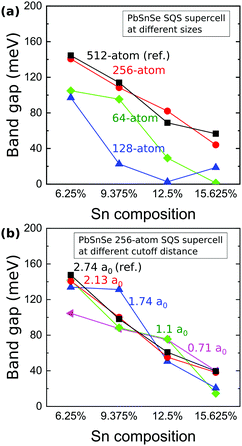Fig. 7 Convergence test for (PbSe)1−x(SnSe)x SQS supercells. Band gap at L point (a) when increasing the size of SQS supercell, and (b) when increasing the distance cutoff of 2- and 3-body correlation functions in 256-atom SQS supercell. In (a) the gaps from 512-atom SQS supercells are chosen as references (‘ref.’), while in (b) the gaps from 2.74 a0 (lattice constant) cutoff distance are chosen as references.

For the study of WSM phase and Weyl points, we constructed different supercells with target symmetries by (1) first generating multiple SQS supercells with given size then (2) picking up the cells that have target symmetries. For each row in Table 1 we calculated one supercell, changing its lattice constant continuously, as an order parameter to tune the band inversion. The searching of Weyl points and the calculation of chirality of Weyl points are then done in the WannierTools code. The flow chart of how we recognize different topological phases is shown in Fig. 8.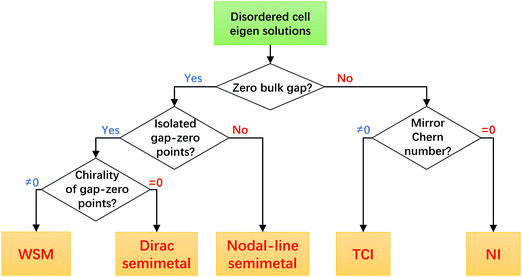Fig. 8 The flow chart for the recognition of topological phases.

### Effective band structure

The basic concept of EBS can be described using the following equations. Assume in the supercell |Km〉 is the m-th electronic eigen state at K in supercell BZ whereas in a primitive cell |kin〉 is the n-th eigen state at ki in primitive BZ, then each |Km〉 can be expanded on a complete set of |kin〉 where K = kiGi, and Gi being reciprocal lattice vectors in the supercell BZ, which is the folding mechanism26
 (1)

The supercell band structure at K can then be unfolded back to ki by calculating the spectral weight PKm(ki)

 (2)

PKm(ki) represents ‘how much’ Bloch characteristics of wavevector ki has been preserved in |Km〉 when En = Em. The EBS is then calculated by spectral function A(ki,E)

 (3)

## Conflicts of interest

The authors declare that there are no conflicts of interest related to this work.

## Acknowledgements

The work at the University of Colorado at Boulder was supported by the National Science foundation NSF Grant NSF-DMR-CMMT No. DMR-1724791. Q. L. was supported by the National Natural Science Foundation of China (NSFC) under Grant Number 11874195. J.-W. L. was supported by the National Natural Science Foundation of China (NSFC) under Grant Number 61888102. The ab initio calculations were done using the Extreme Science and Engineering Discovery Environment (XSEDE), which is supported by the National Science Foundation grant number ACI-1548562. We thank Dr Quansheng Wu for fruitful discussions.

## References

1. C. L. Kane and E. J. Mele, Phys. Rev. Lett., 2005, 95, 146802 CrossRef CAS PubMed.
2. L. Fu, C. L. Kane and E. J. Mele, Phys. Rev. Lett., 2007, 98, 106803 CrossRef PubMed.
3. L. Fu, Phys. Rev. Lett., 2011, 106, 106802 CrossRef PubMed.
4. C.-X. Liu, R.-X. Zhang and B. K. VanLeeuwen, Phys. Rev. B: Condens. Matter Mater. Phys., 2014, 90, 085304 CrossRef.
5. Z. Wang, A. Alexandradinata, R. J. Cava and B. A. Bernevig, Nature, 2016, 532, 189 CrossRef CAS PubMed.
6. S. M. Young, S. Zaheer, J. C. Y. Teo, C. L. Kane, E. J. Mele and A. M. Rappe, Phys. Rev. Lett., 2012, 108, 140405 CrossRef CAS PubMed.
7. T. Zhang, Y. Jiang, Z. Song, H. Huang, Y. He, Z. Fang, H. Weng and C. Fang, Nature, 2019, 566, 475 CrossRef CAS PubMed.
8. M. G. Vergniory, L. Elcoro, C. Felser, N. Regnault, B. A. Bernevig and Z. Wang, Nature, 2019, 566, 480 CrossRef CAS PubMed.
9. F. Tang, H. C. Po, A. Vishwanath and X. Wan, Nature, 2019, 566, 486 CrossRef CAS PubMed.
10. R. S. K. Mong, A. M. Essin and J. E. Moore, Phys. Rev. B: Condens. Matter Mater. Phys., 2010, 81, 245209 CrossRef.
11. M. M. Otrokov, I. P. Rusinov, M. Blanco-Rey, M. Hoffmann, A. Yu. Vyazovskaya, S. V. Eremeev, A. Ernst, P. M. Echenique, A. Arnau and E. V. Chulkov, Phys. Rev. Lett., 2019, 122, 107202 CrossRef CAS PubMed.
12. A. Zunger, Nature, 2019, 566, 447 CrossRef CAS PubMed.
13. L. Fu and C. L. Kane, Phys. Rev. B: Condens. Matter Mater. Phys., 2007, 76, 045302 CrossRef.
14. S. Murakami, New J. Phys., 2007, 9, 356 CrossRef.
15. D. Hsieh, D. Qian, L. Wray, Y. Xia, Y. S. Hor, R. J. Cava and M. Z. Hasan, Nature, 2008, 452, 970–974 CrossRef CAS PubMed.
16. P. Dziawa, B. J. Kowalski, K. Dybko, R. Buczko, A. Szczerbakow, M. Szot, E. Łusakowska, T. Balasubramanian, B. M. Wojek, M. H. Berntsen, O. Tjernberg and T. Story, Nat. Mater., 2012, 11, 1023–1027 CrossRef CAS PubMed.
17. Y. Okada, M. Serbyn, H. Lin, D. Walkup, W. Zhou, C. Dhital, M. Neupane, S. Xu, Y. J. Wang, R. Sankar, F. Chou, A. Bansil, M. Z. Hasan, S. D. Wilson, L. Fu and V. Madhavan, Science, 2013, 341, 1496–1499 CrossRef CAS PubMed.
18. A. Narayan, D. Di Sante, S. Picozzi and S. Sanvito, Phys. Rev. Lett., 2014, 113, 256403 CrossRef PubMed.
19. T.-R. Chang, S.-Y. Xu, G. Chang, C.-C. Lee, S.-M. Huang, B. Wang, G. Bian, H. Zheng, D. S. Sanchez, I. Belopolski, N. Alidoust, M. Neupane, A. Bansil, H.-T. Jeng, H. Lin and M. Zahid Hasan, Nat. Commun., 2016, 7, 10639 CrossRef CAS PubMed.
20. L. Nordheim, Ann. Phys., 1931, 9, 607 CrossRef CAS.
21. C. Yan, J. Liu, Y. Zang, J. Wang, Z. Wang, P. Wang, Z.-D. Zhang, L. Wang, X. Ma and S. Ji, Phys. Rev. Lett., 2014, 112, 186801 CrossRef PubMed.
22. P. Soven, Phys. Rev., 1967, 156, 809–813 CrossRef CAS.
23. D. Di Sante, P. Barone, E. Plekhanov, S. Ciuchi and S. Picozzi, Sci. Rep., 2015, 5, 11285 CrossRef CAS PubMed.
24. L.-W. Wang, L. Bellaiche, S.-H. Wei and A. Zunger, Phys. Rev. Lett., 1998, 80, 4725 CrossRef CAS.
25. V. Popescu and A. Zunger, Phys. Rev. Lett., 2010, 104, 236403 CrossRef PubMed.
26. V. Popescu and A. Zunger, Phys. Rev. B: Condens. Matter Mater. Phys., 2012, 85, 085201 CrossRef.
27. Z. Wang, J.-W. Luo and A. Zunger, Phys. Rev. Mater., 2019, 3, 044605 CrossRef CAS.
28. A. A. Burkov and L. Balents, Phys. Rev. Lett., 2011, 107, 127205 CrossRef CAS PubMed.
29. H. Weng, C. Fang, Z. Fang, B. A. Bernevig and X. Dai, Phys. Rev. X, 2015, 5, 011029 Search PubMed.
30. B. Q. Lv, H. M. Weng, B. B. Fu, X. P. Wang, H. Miao, J. Ma, P. Richard, X. C. Huang, L. X. Zhao, G. F. Chen, Z. Fang, X. Dai, T. Qian and H. Ding, Phys. Rev. X, 2015, 5, 031013 Search PubMed.
31. S.-Y. Xu, I. Belopolski, N. Alidoust, M. Neupane, G. Bian, C. Zhang, R. Sankar, G. Chang, Z. Yuan, C.-C. Lee, S.-M. Huang, H. Zheng, J. Ma, D. S. Sanchez, B. Wang, A. Bansil, F. Chou, P. P. Shibayev, H. Lin, S. Jia and M. Z. Hasan, Science, 2015, 349, 613–617 CrossRef CAS PubMed.
32. S. Murakami, M. Hirayama, R. Okugawa and T. Miyake, Sci. Adv., 2017, 3, e1602680 CrossRef PubMed.
33. A. J. Strauss, Phys. Rev., 1967, 157, 608 CrossRef CAS.
34. F. Orbanić, M. Novak, S. Pleslić and I. Kokanović, J. Phys.: Conf. Ser., 2018, 969, 012142 CrossRef.
35. B. Bradlyn, J. Cano, Z. Wang, M. G. Vergniory, C. Felser, R. J. Cava and B. A. Bernevig, Science, 2016, 353, 6299 CrossRef PubMed.
36. M. Neupane, S.-Y. Xu, R. Sankar, Q. Gibson, Y. J. Wang, I. Belopolski, N. Alidoust, G. Bian, P. P. Shibayev, D. S. Sanchez, Y. Ohtsubo, A. Taleb-Ibrahimi, S. Basak, W.-F. Tsai, H. Lin, T. Durakiewicz, R. J. Cava, A. Bansil, F. C. Chou and M. Z. Hasan, Phys. Rev. B: Condens. Matter Mater. Phys., 2015, 92, 075131 CrossRef.
37. J. C. Mikkelsen and J. B. Boyce, Phys. Rev. B: Condens. Matter Mater. Phys., 1983, 28, 7130–7140 CrossRef CAS.
38. Q. T. Islam and B. A. Bunker, Phys. Rev. Lett., 1987, 59, 2701 CrossRef CAS PubMed.
39. B. A. Bunker, Z. Wang and Q. Islam, Ferroelectrics, 1993, 150, 171–182 CrossRef.
40. I.-K. Jeong, T. W. Darling, J. K. Lee, Th. Proffen, R. H. Heffner, J. S. Park, K. S. Hong, W. Dmowski and T. Egami, Phys. Rev. Lett., 2005, 94, 147602 CrossRef PubMed.
41. S. Kastbjerg, N. Bindzus, M. Søndergaard, S. Johnsen, N. Lock, M. Christensen, M. Takata, M. A. Spackman and B. B. Iversen, Adv. Funct. Mater., 2013, 23, 5477–5483 CrossRef CAS.
42. J. L. Martins and A. Zunger, Phys. Rev. B: Condens. Matter Mater. Phys., 1984, 30, 6217–6220 CrossRef CAS.
43. E. S. Božin, C. D. Malliakas, P. Souvatzis, T. Proffen, N. A. Spaldin, M. G. Kanatzidis and S. J. L. Billinge, Science, 2010, 330, 1660–1663 CrossRef PubMed.
44. A. N. Beecher, O. E. Semonin, J. M. Skelton, J. M. Frost, M. W. Terban, H. Zhai, A. Alatas, J. S. Owen, A. Walsh and S. J. L. Billinge, ACS Energy Lett., 2016, 1, 880–887 CrossRef CAS.
45. C. W. Groth, M. Wimmer, A. R. Akhmerov, J. Tworzyd\lo and C. W. J. Beenakker, Phys. Rev. Lett., 2009, 103, 196805 CrossRef CAS PubMed.
46. J. Li, R.-L. Chu, J. K. Jain and S.-Q. Shen, Phys. Rev. Lett., 2009, 102, 136806 CrossRef PubMed.
47. A. Zunger, S.-H. Wei, L. G. Ferreira and J. E. Bernard, Phys. Rev. Lett., 1990, 65, 353 CrossRef CAS PubMed.
48. S.-H. Wei, L. G. Ferreira, J. E. Bernard and A. Zunger, Phys. Rev. B: Condens. Matter Mater. Phys., 1990, 42, 9622–9649 CrossRef CAS PubMed.
49. J. Liu, C. Fang and L. Fu, 2016, arXiv:1604.03947v1.
50. J. Liu and D. Vanderbilt, Phys. Rev. B: Condens. Matter Mater. Phys., 2014, 90, 155316 CrossRef.
51. A. Łusakowski, P. Bogusławski and T. Story, Phys. Rev. B, 2018, 98, 125203 CrossRef.
52. G. Kresse, http://www.vasp.at.
53. A. A. Mostofi, J. R. Yates, G. Pizzi, Y.-S. Lee, I. Souza, D. Vanderbilt and N. Marzari, Comput. Phys. Commun., 2014, 185, 2309–2310 CrossRef CAS.
54. Q. Wu, S. Zhang, H.-F. Song, M. Troyer and A. A. Soluyanov, Comput. Phys. Commun., 2018, 224, 405–416 CrossRef CAS.
55. P. V. C. Medeiros, S. Stafström and J. Björk, Phys. Rev. B: Condens. Matter Mater. Phys., 2014, 89, 041407 CrossRef.
56. S. L. Dudarev, G. A. Botton, S. Y. Savrasov, C. J. Humphreys and A. P. Sutton, Phys. Rev. B: Condens. Matter Mater. Phys., 1998, 57, 1505 CrossRef CAS.
57. S.-H. Wei and A. Zunger, Phys. Rev. B: Condens. Matter Mater. Phys., 1997, 55, 13605 CrossRef CAS.
58. A. Szczerbakow and H. Berger, J. Cryst. Growth, 1994, 139, 172–178 CrossRef CAS.
 This journal is © The Royal Society of Chemistry 2019